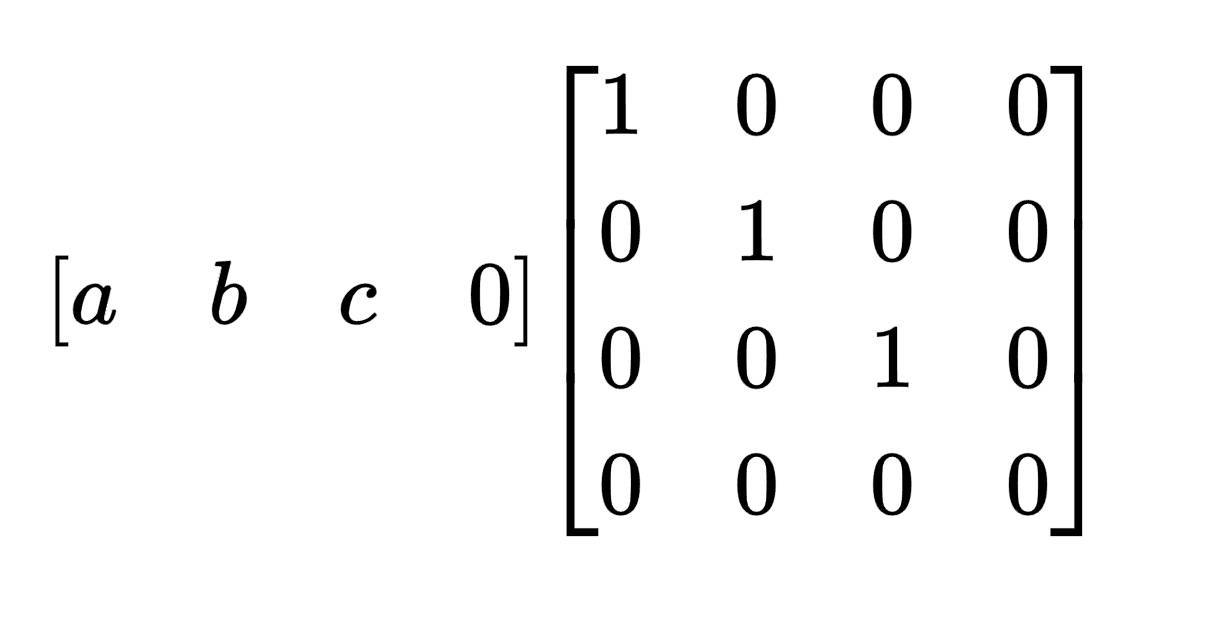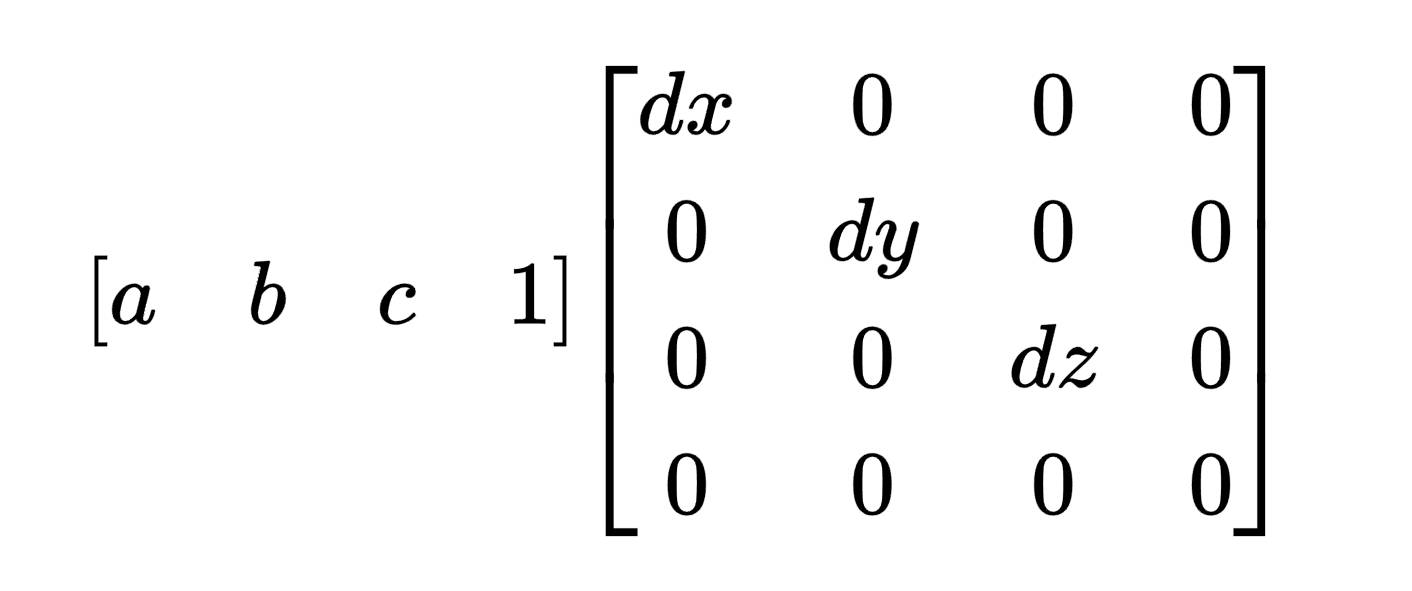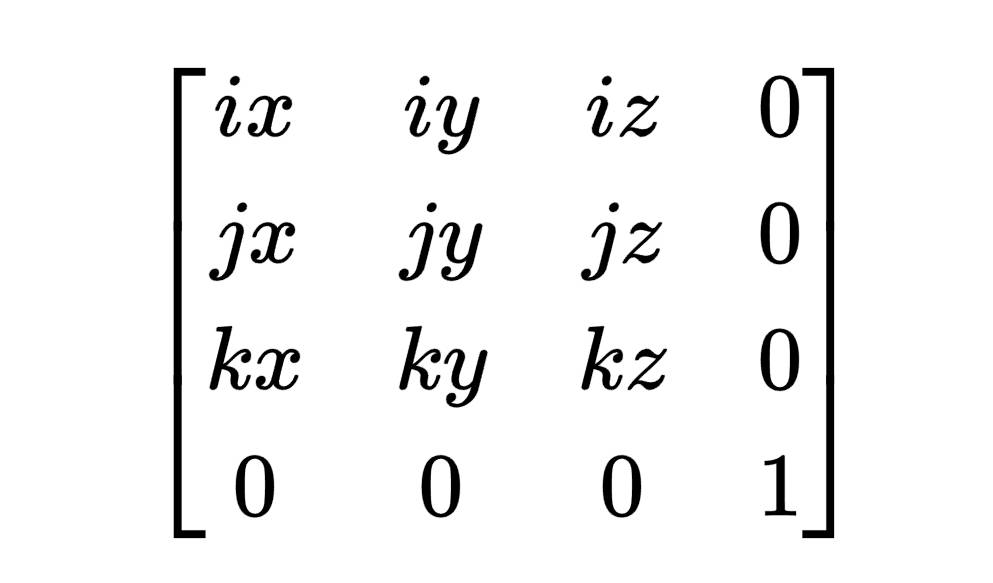# 坐标系、点和向量的矩阵表示 #

## 向量和点的矩阵表示 #

### （1）向量的矩阵表示 #

``````v1 = a * i + b * j + c * k; // i, j, k 为 x，y，z 轴方向的基向量
// 加上一个零向量
v1 = a * i + b * j + c * k + 0 * o; // o 表示零向量
``````### （2）点的矩阵表示 #

``````p1 = 1 * o + a * d.x + b * d.y + c * d.z; // o 表示坐标系原点，d 表示移动向量
````````````// 点和向量的加法，向量 (1,1,1) 与点 (1,1,1)，结果最后一位为 1 表示点
(1, 1, 1, 1) + (1, 1, 1, 0) = (2, 2, 2, 1)
// 向量和向量的加法，结果最后一位为 0 表示向量
(1, 1, 1, 0) + (1, 1, 1, 0) = (2, 2, 2, 0)
...
``````

## 坐标系的矩阵表示 #（完）